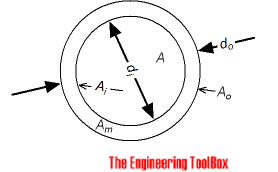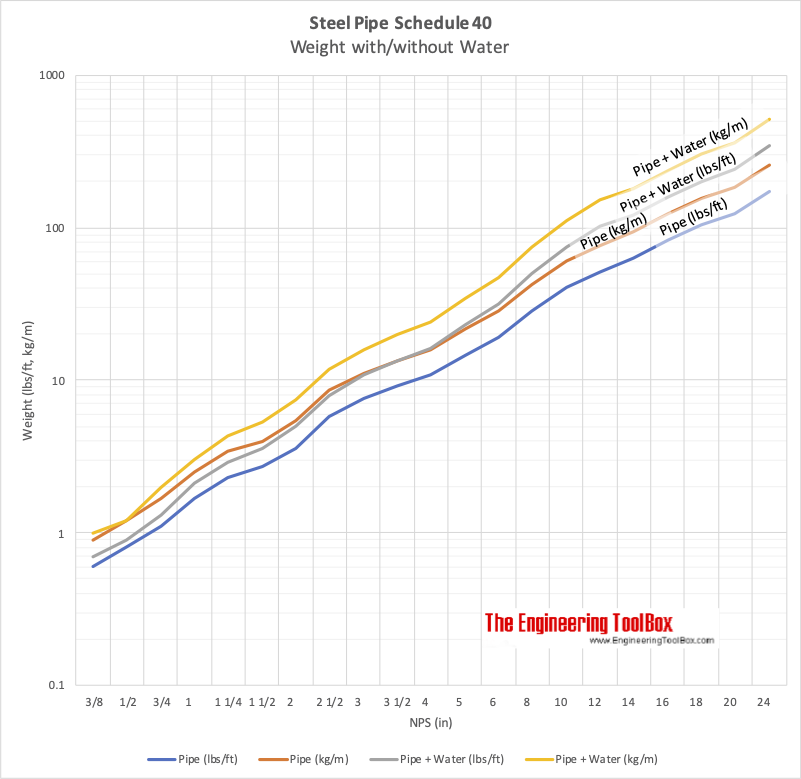Engineering ToolBox - Resources, Tools and Basic Information for Engineering and Design of Technical Applications!

# Pipe Weight Calculator

## Weight calculating equation for steel pipes.### Weight of Empty Pipe

Weight of empty pipe per unit length can be calculated as

wpρm Am

= ρm π (do2 - di2) / 4

= (π / 4) ρm (do2 - di2)                               (1)

where

wpweight of empty pipe per unit length (kg/m, lb/in)

ρm = density of pipe material (kg/m3, lb/in3)

Am = cross-sectional wall area of pipe (m2, in2)

do = outside diameter (m, in)

di = do - 2 t = inside diameter (m, in)

t = wall thickness (m, in)

### Weight of Liquid in Pipe

Weight of liquid in pipes per unit length can be calculated as

wl = ρl Ai

= ρl π (di / 2)2

= (π / 4) ρl di2                                    (2)

where

wl = weight of liquid in pipe per unit length of pipe (kg, lb)

Ai = cross-sectional inside area of pipe (m2, in2)

ρl = density of liquid (kg/m3, lb/in3)

### Weight of Pipe with Liquid

Weight of pipe with liquid can be calculated as

w = wp + wi

= ρm Am + ρl Ai

=  (ρm π (do2 - di2) / 4) + (ρl π di2 / 4)

= (π / 4) [ρm (do2 - di2) + ρl di2]                      (3)

#### Weight of Pipe Calculator

This calculator can be used to calculate the weight of a pipe with - or without - liquid. The calculator is generic and can be used for both SI and Imperial units as long as the use of units are consistent.

do - outside diameter (m, in)

di - inside diameter (m, in)

ρm - density of piping material (kg/m3, lb/in3)

ρldensity of liquid (kg/m3, lb/in3) (zero for empty pipe)

• 1 m = 103 mm
• 1 m2 = 106 mm2
• 1 in = 1/12 ft
• 1 in2 = 1/144 ft2
• 1 lb/in3 = 1728 lb/ft2

### Example - Weight of 4" Schedule 40 Steel Pipe with Water - SI Units (default values in the calculator above)

The outside diameter  of 4" Schedule 40 Steel Pipe is 114.3 mm. The inside diameter is 102.3 mm. The density of steel is 7850 kg/m3. The density of water is 1000 kg/m3.

The weight of empty pipe per unit length can be calculatet with (1) as:

wp = (π / 4) (7850 kg/m3) ((0.1143 m)2 - (0.1023 m)2)

= 16 kg/m

The weight of the liquid in the pipe per unit length can be calculatet with (2) as:

wl = (π / 4) (1000 kg/m3) (0.1023 m)2

= 8.2 kg/m

The weight of pipe filled with water per unit length can be calculated with (3) as

w = (π / 4) [(7850 kg/m3)((0.1143 m)2 - (0.1023 m)2) + (1000 kg/m3) (0.1023 m)2

= 24.2 kg/m

### Example - Weight of 4" Schedule 40 Steel Pipe

The outside diameter  of 4" Schedule 40 Steel Pipe is 4.500 inches. The wall thickness is 0.237 inches and the inside diameter is 4.026 in. The density of steel is 490 lb/ft3 (0.28 lb/in3). The weight of empty pipe per unit length can be calculated as

w = (π / 4) (0.28 lb/in3) ((4.500 in)2 - (4.026 in)2)

= 0.89 lb/in

= 10.7 lb/ft

### Typical Weight of Schedule 40 Steel Pipe with/without Water

Nominal Pipe SizeWeight of PipeWeight of Pipe filled with Water
(in)(mm)(lbs/ft)(kg/m)(lbs/ft)(kg/m)
3/8 10 0.6 0.9 0.7 1.0
1/2 15 0.8 1.2 0.9 1.2
3/4 20 1.1 1.7 1.3 2.0
1 25 1.7 2.5 2.1 3.0
1 1/4 32 2.3 3.4 2.9 4.3
1 1/2 40 2.7 4.0 3.6 5.3
2 50 3.6 5.4 5.0 7.5
2 1/2 65 5.8 8.6 7.9 11.7
3 80 7.6 11.2 10.8 15.9
3 1/2 90 9.1 13.5 13.4 19.8
4 100 10.8 16.0 16.3 24.2
5 125 14.6 21.7 23.2 34.6
6 150 19.0 28.2 31.5 46.8
8 200 28.5 42.5 50.1 74.6
10 250 40.5 60.2 74.6 111
12 300 51.1 75.9 102 152
14 350 63.0 93.7 122 181
16 400 83.0 124 160 237
18 450 105 156 202 301
20 500 123 183 243 362
24 600 171 255 345 514### Weight of Insulation on Pipe

Weight of stone wool insulation mat on pipe.

Nominal DiameterOutside Diameter
(mm)
Weight of Insulation (kg/m pipe)
Insulation Thichness (mm)
(mm)(in)3040506080100120140
15 ½ 21.3 4 5 6 8 11 15 19 24
25 1 33.7 4 5 7 8 12 15 20 25
50 2 60.3 5 7 8 10 13 17 22 27
65 76.1 6 7 9 10 14 18 23 28
80 3 88.9 7 8 10 11 15 19 24 29
100 4 114.3 8 9 11 12 16 21 26 31
200 8 219.1 12 14 16 18 23 28 33 39
300 12 323.9 17 19 21 24 29 35 41 47
500 20 508.0 25 28 31 34 40 47 54 62
700 28 711.0 34 37 41 44 52 60 69 78

## Related Topics

• Dimensions - Sizes and dimensions of pipes and tubes, and their fittings - inside and outside diameter, weight and more.

## Engineering ToolBox - SketchUp Extension - Online 3D modeling!

Add standard and customized parametric components - like flange beams, lumbers, piping, stairs and more - to your Sketchup model with the Engineering ToolBox - SketchUp Extension - enabled for use with the amazing, fun and free SketchUp Make and SketchUp Pro .Add the Engineering ToolBox extension to your SketchUp from the SketchUp Pro Sketchup Extension Warehouse!

Translate

## Privacy

We don't collect information from our users. Only emails and answers are saved in our archive. Cookies are only used in the browser to improve user experience.

Some of our calculators and applications let you save application data to your local computer. These applications will - due to browser restrictions - send data between your browser and our server. We don't save this data.

## Citation

• Engineering ToolBox, (2004). Pipe Weight Calculator. [online] Available at: https://www.engineeringtoolbox.com/pipe-weight-calculation-d_745.html [Accessed Day Mo. Year].

Modify access date.

. .

#### Scientific Online Calculator6 24